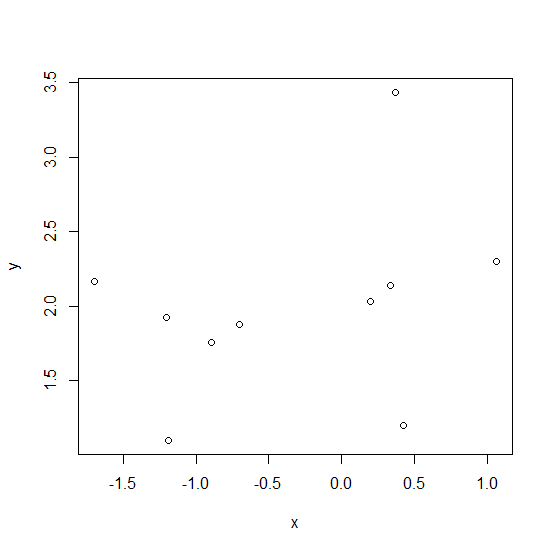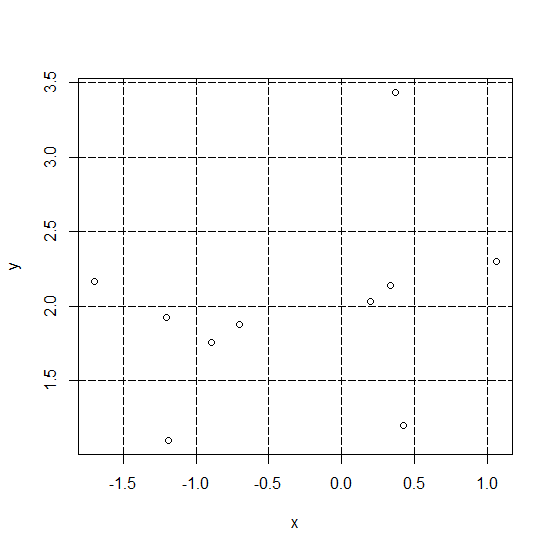# How to draw gridlines in a graph with abline function in R?

R ProgrammingServer Side ProgrammingProgramming

Gridlines are the horizontal and vertical dotted lines, and they help to organize the chart so that the values on the labels becomes better readable to viewers. This is helpful specially in situations where we plot a large number of data points. A graph drawn by plot function can have gridlines by defining the vertical and horizontal lines using abline.

## Example

Consider the below data and scatterplot −

Live Demo

> x<-rnorm(10)
> y<-rnorm(10,1.5)
> plot(x,y)

## OutputAdding gridlines using abline function −

> abline(h=seq(0,5,0.5),lty=5)
> abline(v=seq(-2,2,0.5),lty=5)

## Output